Next: Multiplication Up: Dyadic Stream Operations Previous: Dyadic Stream Operations

## Average

Computing the average two streams of dyadic digits x and y can be performed using a relatively simple recursive algorithm. Here we examine the first digit of each of these two input streams, and then use the properties given in section 3.6.2 to derive such an algorithm which returns their average as a new dyadic digit stream.

Suppose x is of the form (a :: x') and y is of the form (b :: y'). We use the observation that a prefixing a digit to a stream using the cons' operation may be interpreted as the average of the numbers represented by the digit and the stream, and then apply the exchange law to say:

Note that in the third equation the term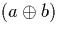is the average of two dyadic digits, and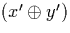is the average of two streams. Dyadic digits are closed under average, and hence the number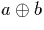can represented in a single dyadic digit. If we do this, the average of these two terms will be of a single dyadic digit and a dyadic digit stream. We use this fact, and the property that a digit averaged with a stream can be implemented by using the cons' operation to prefix the digit to the stream, to give us the following: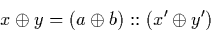This leads to a simple recursive algorithm, expressed here as the function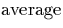, for computing dyadic stream average. It makes use of the algorithm for computing the average of two dyadic digits,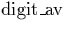, which is described in section A.2.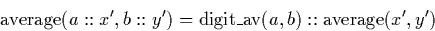The algorithm is convergent because it need examine only one digit of each input stream to generate a digit of output. The performance of this algorithm is examined further in section 7.1.2.Next: Multiplication Up: Dyadic Stream Operations Previous: Dyadic Stream Operations
Martin Escardo
5/11/2000